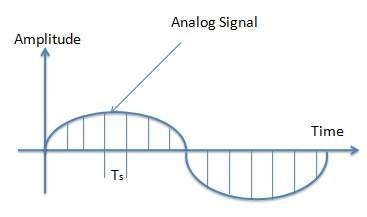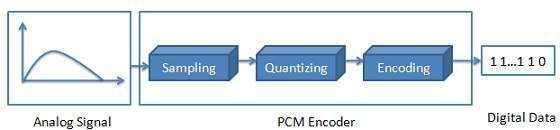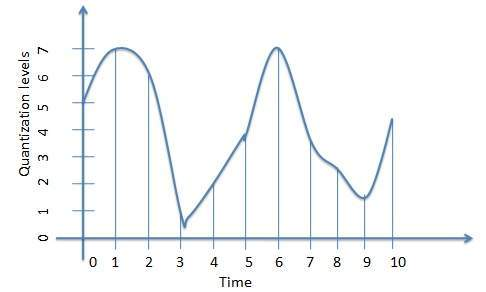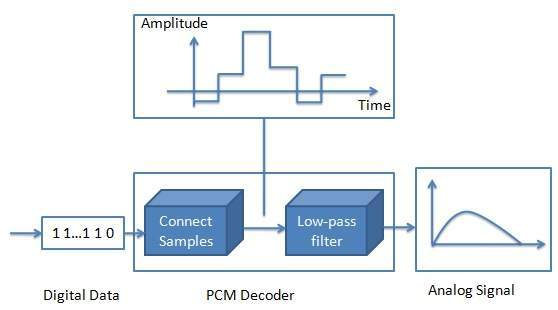# Multimedia Sound & Audio

## Digitization of Sound

Digitization is a process of converting the analog signals to a digital signal. There are three steps of digitization of sound.• Sampling - Sampling is a process of measuring air pressure amplitude at equally spaced moments in time, where each measurement constitutes a sample. A sampling rate is the number of times the analog sound is taken per second. A higher sampling rate implies that more samples are taken during the given time interval and ultimately, the quality of reconstruction is better. The sampling rate is measured in terms of Hertz, Hz in short, which is the term for Cycle per second. A sampling rate of 5000 Hz(or 5kHz,which is more common usage) implies that mt uj vu8i 9ikuhree sampling rates most often used in multimedia are 44.1kHz(CD-quality), 22.05kHz and 11.025kHz.

• Quantization - Quantization is a process of representing the amplitude of each sample as integers or numbers. How many numbers are used to represent the value of each sample known as sample size or bit depth or resolution. Commonly used sample sizes are either 8 bits or 16 bits. The larger the sample size, the more accurately the data will describe the recorded sound. An 8-bit sample size provides 256 equal measurement units to describe the level and frequency of the sound in that slice of time. A 16-bit sample size provides 65,536 equal units to describe the sound in that sample slice of time. The value of each sample is rounded off to the nearest integer (quantization) and if the amplitude is greater than the intervals available, clipping of the top and bottom of the wave occurs.

• Encoding - Encoding converts the integer base-10 number to a base-2 that is a binary number. The output is a binary expression in which each bit is either a 1(pulse) or a 0(no pulse).## Quantization of Audio

Quantization is a process to assign a discrete value from a range of possible values to each sample. Number of samples or ranges of values are dependent on the number of bits used to represent each sample. Quantization results in stepped waveform resembling the source signal.

• Quantization Error/Noise - The difference between sample and the value assigned to it is known as quantization error or noise.

• Signal to Noise Ratio (SNR) - Signal to Ratio refers to signal quality versus quantization error. Higher the Signal to Noise ratio, the better the voice quality. Working with very small levels often introduces more error. So instead of uniform quantization, non-uniform quantization is used as companding. Companding is a process of distorting the analog signal in controlled way by compressing large values at the source and then expanding at receiving end before quantization takes place.## Transmission of Audio

In order to send the sampled digital sound/ audio over the wire that it to transmit the digital audio, it is first to be recovered as analog signal. This process is called de-modulation.

• PCM Demodulation - PCM Demodulator reads each sampled value then apply the analog filters to suppress energy outside the expected frequency range and outputs the analog signal as output which can be used to transmit the digital signal over the network.Print